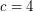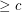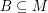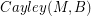tension

5-local-tensions ★★

Author(s): DeVos

Conjecture   There exists a fixed constant(probablysuffices) so that every embedded (loopless) graph with edge-widthhas a 5-local-tension.

Keywords: coloring; surface; tension

A homomorphism problem for flows ★★

Author(s): DeVos

Conjecture   Letbe abelian groups and letandsatisfyand. If there is a homomorphism fromto, then every graph with a B-flow has a B'-flow.

Keywords: homomorphism; nowhere-zero flow; tension